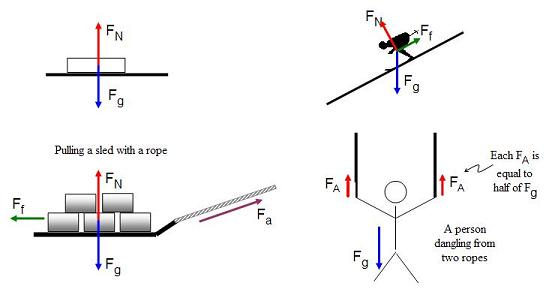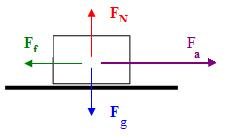Lesson 19: Net Force

Up to this point we have been focusing on situations that involve only one force acting on an object.

• In the real world it is rare to actually find situations like this.
• Usually there will be at least a couple of forces acting on an object.
• What we need to do is figure out how all these individual forces act on the object overall.

This is what we do when we look at net force.

• When someone talks about his gross pay and net pay, what do they mean?
• Gross pay is how much you are paid before any deductions.
• Net pay is how much you actually get on your paycheck after all the deductions.
• It’s the same sort of thing when we examine net force.
• After you have added and subtracted all the forces you are left with the net force acting on the object.

There are several common forces acting on objects that you need to memorize:

Fg = force due to gravity

Fa = applied force

FN = normal force

FNET = net force

Ff = force due to friction

To keep track of how all these forces are affecting a single object, it is a good idea to draw a free body diagram.

• A free body diagram is just a simple sketch of the object showing all the forces that are acting on it.
1. Draw a quick sketch of the object.
2. Draw an arrow showing every force acting on the object.
3. To calculate the net force, add any vectors acting on the same axis (x and y), making sure to pay attention to the directions.Quite often we are able to ignore many of the forces that cancel each other out.

• We focus on the forces that are actually involved in how the object will move.
• Most of the time the two forces we can focus on are Fa and Ff.
• When we calculate the net force acting on the object in these situations, we use the formula…

FNET = Fa + Ff

• Notice that we don’t say we are subtracting the two forces.
• Instead, it’s up to you to remember that if one of the forces is pointing in one direction (like to the right), and the other force is pointing in the other direction (like to the left), you will need to make one positive and the other negative.

Example 1: I want to push my tarantula’s 8.7kg cage across the table. I push with 29N of force, and there is a force due to friction of 8N between the table and the cage. Determine how much the cage will accelerate.

First, draw a free body diagram.Since nothing is happening along the y-axis, we can ignore the Fg and FN forces.

FNET = Fa + Ff

= 29N + -8N

FNET = 21N

I had to make the friction a negative force because it is pointing in the direction opposite to the applied force.

When you want to calculate the acceleration of an object, always use the net force acting on it.

FNET = ma

a = FNET / m

= (21N) / (8.7kg)

a = 2.4 m/s2

## The Elevator Question

The concept of net force becomes a bit more complicated when you examine a complex system like an elevator going up and down.

• It might sound strange to call an elevator complex, but it really does make a challenging problem.
• How do you think you would solve a question that asks you about your weight as an elevator accelerates up or down?

When the elevator is accelerating up, what would happen to your weight?

• Have you ever noticed that when an elevator first starts to move up, you feel yourself pushed down a little?
• This is because you can feel the elevator’s acceleration… it basically makes you feel a bit heavier for a moment.
• A scale would show this as an increase in your weight (temporarily).

What would happen to your apparent weight if the elevator started to accelerate down?

• You would feel the elevator drop out underneath you.
• If it really dropped out underneath you, it would feel just like being on the “Space Shot ” at Galaxyland as it is falling … you’d feel weightless!
• A scale would show your weight decreasing.

Let’s look at how we would actually figure out some numbers for this type of question by looking at an example.

• Keep one thing in the back of your mind. A regular scale is made to measure things in kilograms, and it is built for Earth’s regular gravity of 9.81m/s2. You’ll see why this is important later.

Example 2: You are standing on a scale in an elevator. You have a mass of 75kg. Determine what a scale would show as your “apparent” mass (in kilograms) if…

a) the elevator starts to accelerate upwards at 3.0m/s2.

We need to think of the net force as the force pushing down on the scale causing it to give a reading.

• The elevator pushing up on me will make me push down on the floor (Newton’s 3rd Law).
• We will show this by making the acceleration (3.0m/s2) a negative value.
• The formula for this would look like this…

FNET = Fg + Fa

• We will treat the force due to gravity (Fg) as just the regular force of my body pushing down on the elevator's floor.
• The applied force (Fa) is how much the acceleration of the elevator is increasing or decreasing my force on the elevator's floor.

FNET = Fg + Fa

= mg + ma <- since mass is common, I’ll factor it out

= m (g + a)

= 75kg (-9.81m/s2 + -3.0m/s2) <- both are negative

= 75kg (-12.81m/s2)

FNET = -9.6e2 N

• Oy! When was the last time you saw a scale give a reading in Newtons?
• I can change this into a reading in kilograms by remembering that the scale we're using has no idea what is going on... it still thinks it's sitting in someone's bathroom where gravity is a nice constant 9.81m/s2.

FNET = mg

m = FNET / g

= (-9.6e2 N) / (-9.81m/s2)

m = 98 kg

So a regular scale thinks you weigh 98 kg!

b) the elevator starts to accelerate downwards at 4.0m/s2.

We’ll handle this part of the question the same way.

• The only difference is that because the elevator is dropping out from under me, I will push less against the floor.
• The acceleration of the elevator will be positive.

FNET = Fg + Fa

= mg + ma

= m (g + a)

= 75kg (-9.81m/s2 + 4.0m/s2) <- both are negative

= 75kg (-5.81m/s2)

FNET = -4.4e2 N

The scale will read...

FNET = mg

m = FNET / g

= (-4.4e2 N) / (-9.81m/s2)

m = 44 kg

So a regular scale thinks you weigh 44 kg!